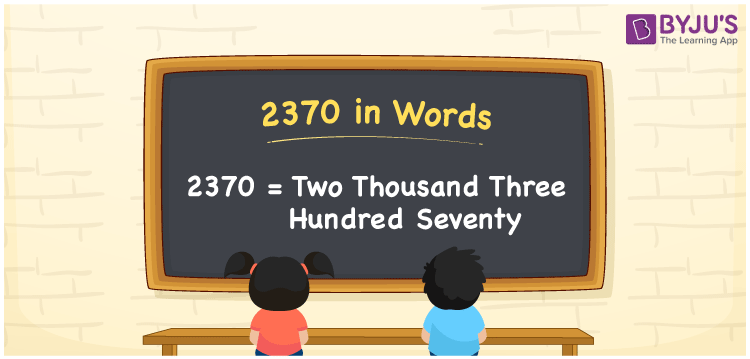# 2370 in Words

We can write 2370 in words as Two thousand three hundred seventy. It is easy to transform the given number, i.e. 2370, into words with the help of a place value chart. Also, we can observe that the word forms of cardinal numbers are used in general conversations. Suppose you purchased a painting kit that costs Rs. 2370, you can say, “I purchased a painting kit for Two thousand three hundred seventy rupees”. In the same way, we use the word form of 2370 in many conversations.

 2370 in words Two thousand three hundred seventy Two thousand three hundred seventy in Numbers 2370

## 2370 in English Words

Using the English alphabet, we can express numbers in words. Thus, we can spell 2370 in words as “Two thousand three hundred seventy”.## How to Write 2370 in Words?

The below table illustrates the place value chart for the number 2370. Using the place values defined below, we can convert the number 2370 into words.

 Thousands Hundreds Tens Ones 2 3 7 0

Here, ones = 0, tens = 7, hundreds = 3, and thousands = 2.

By expanding these numbers, we get;

2 × Thousand + 3 × Hundred + 7 × Ten + 0 × One

= 2 × 1000 + 3 × 100 + 7 × 10 + 0 × 1

= 2000 + 300 + 70

= Two thousand + Three hundred + Seventy

= Two thousand three hundred seventy

Therefore, 2370 in words = Two thousand three hundred seventy.

Learn in detail about place value here.

2370 is a natural number that precedes 2371 and succeeds 2369.

2370 in words – Two thousand three hundred seventy

Is 2370 an odd number? – No

Is 2370 an even number? – Yes

Is 2370 a prime number? – No

Is 2370 a composite number? – Yes

Is 2370 a perfect square number? – No

Is 2370 a perfect cube number? – No

## Frequently Asked Questions on 2370 in Words

Q1

### What number is Two thousand three hundred seventy in words?

The number 2370 is written as Two thousand three hundred seventy in words.
Q2

### How to write Rs. 2370 in words on a cheque?

On a cheque, we can write Rs. 2370 in words as “Two thousand three hundred seventy rupees only”.
Q3

### Express the value of 2370 + 2741 in words.

2370 + 2741 = 5111 Thus, the value of 2370 + 2741, i.e. 5111 can be expressed in words as Five thousand one hundred eleven.